Next: Parallel partitioning algorithms Up: The State of the Previous: Hybrid approach

### Multilevel approach

The idea of the multilevel approach is to encapsulate the connectivity information of a very large graph (of, say, over 1 million vertices) by a series of coarser and coarser graphs. An algorithm based on the multilevel approach normally has 3 phases

• coarsening phase: the original graph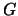is reduced into a series of successively coarser graphs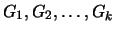.

• partitioning phase: partition the coarsest graph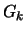into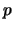parts;
• uncoarsening and refinement phase: the partitioning ofis interpolated to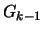and refined, the process repeated and terminated at.

This approach is in a way similar to the multigrid idea for grid based linear equation solvers . The multilevel approach was first introduced  to speedup the recursive spectral bisection (RSB) algorithm, and was soon used in combination with K-L type refinement algorithms [36,47,82].

The coarsening phase -

There are a number of ways to coarsen a graph. In  the maximal independent set of a graph is chosen as the vertices of the coarse graph. An independent set of the graphis a set of its vertices, with no two of the vertices in the set connected by an edge of. An independent set is a maximal independent set if the addition of an extra vertex makes it no longer independent. Figure 9 illustrates a graph of 788 vertices (which is the dual graph of the mesh in Figure 3), with 2 levels of coarse graphs of 332 and 94 vertices respectively, generated using this method.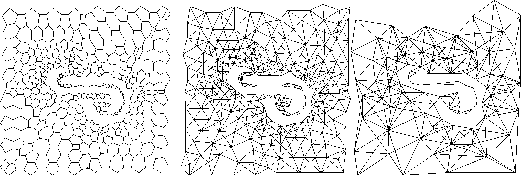The most popular method for generating the multilevel of coarse graphs is based on edge collapsing [36,47]. Selected edges are collapsed so that two vertices connected by one of these edges form a multi-node. Each vertex of the resulting coarse graph has a weight associated with it, which indicates the number of original vertices it contains. Each edge of the coarse graph also has a weight associated with it that indicates the number of original edges it represents. This edge collapsing approach has the distinctive advantage that the edge-cut (generalized here as the sum of the edge weights cut by the partitioning) on the coarsest graph equals the edge-cut of the finest (original) graph, if the partitioning on the coarsest graph were inherited by the finer graphs without further refinement. Therefore to some extent the original problem is encapsulated well by a problem of much smaller size. Figure 10 illustrates the graph of 788 vertices, with 2 levels of coarse graphs of 403 and 210 vertices respectively, generated using this method.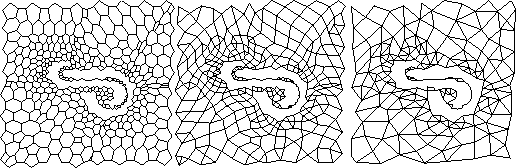The edges are usually selected using the idea of matching. A matching of a graph is a set of edges, such that no two of them share the same vertex. The maximal match is a matching of the largest size. In a heavy edge matching , vertices are visited randomly. For each vertex, the heaviest unmatched edge from the vertex is selected. Heavy edge matching has the advantage that the resulting coarse graph has a relatively small total edge weight, and therefore the partitioning of it is more likely to give a small edge-cut. As an alternative to edge collapsing based on maximal matching, a more aggressive coarsening approach has been employed , where the greedy algorithm (Section 3.2.2) was used to form small clusters of, say, 10 vertices for collapsing. The coarsening process is applied until the graph has less than a preset minimum number of vertices.

The partitioning phase -

As the coarsest graph is of very small size, any partitioning algorithms will be able to partition it rapidly. The partition is executed in a manner such that the summation of the vertex weights of each subgraph should be roughly the same. When edge collapsing approach is used for graph coarsening, the edge-cut of the partitioning is the same as that for the finest graph, should the partitioning be inherited all the way to the finest graph without refinement. The choice of partitioning algorithms at this coarsest level is not very important [47,36] to the overall quality of the partition because refinement will be carried out at the subsequent finer levels of the graph.

The uncoarsening and refinement phase -

During the uncoarsening and refinement phase, the partitioning of a graph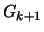is inherited by the finer graph.

For the multilevel spectral bisection (MRSB) algorithm , the eigenvector for the coarse graphis interpolated to the graphas the first approximation of the eigenvector for. From there, instead of using the Lanczos algorithm to recalculate the eigenvector, Rayleigh iterations are used to improve the approximation. The resulting positive semi-definite linear systems are solved using SYMMLQ. Because a good initial approximation exists, the number of matrix-vector products involved in finding the Fiedler vector using the Rayleigh quotient algorithm is normally no more than ten, as opposed to up-to several hundred if the Lanczos algorithm is used (here the matrix refers to the Laplacian matrix of the corresponding graph). Thus the multilevel spectral bisection algorithm is about an order of magnitude faster than the single level Lanczos implementation of RSB.

But there are two disadvantages to the multilevel spectral bisection approach. First, this is still a bisection algorithm and the partitioning of a graph into many subdomains would involve recursive use of the algorithm. Second, even though the Rayleigh quotient procedure is substantially faster than the Lanczos algorithm, each iteration is still of the order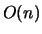, where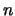is the number of vertices in the graph (but see  for a partial improvement of the situation by contracting the interior of each of the two subdomains into super-nodes). However all that may be needed on the finer graphis some refinement of the subdomain boundary to reduce edge-cut and to maintain the load balance. For a large graph with a good existing partition, the size of the boundary should be only a small fraction of that of the interior. Therefore multilevel algorithms that adopt K-L type algorithms during the uncoarsening and refinement phase have proved themselves to be superior to MRSB [36,82,47]. The boundary refinement approach is not restricted to bisection and-way refinement to the existing partition could be used. In  vertices of any subdomain are allowed to move to any of the other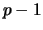subdomains, while in [52,82] boundary vertices are allowed to move to the neighboring subdomains only.Next: Parallel partitioning algorithms Up: The State of the Previous: Hybrid approach

2000-03-21